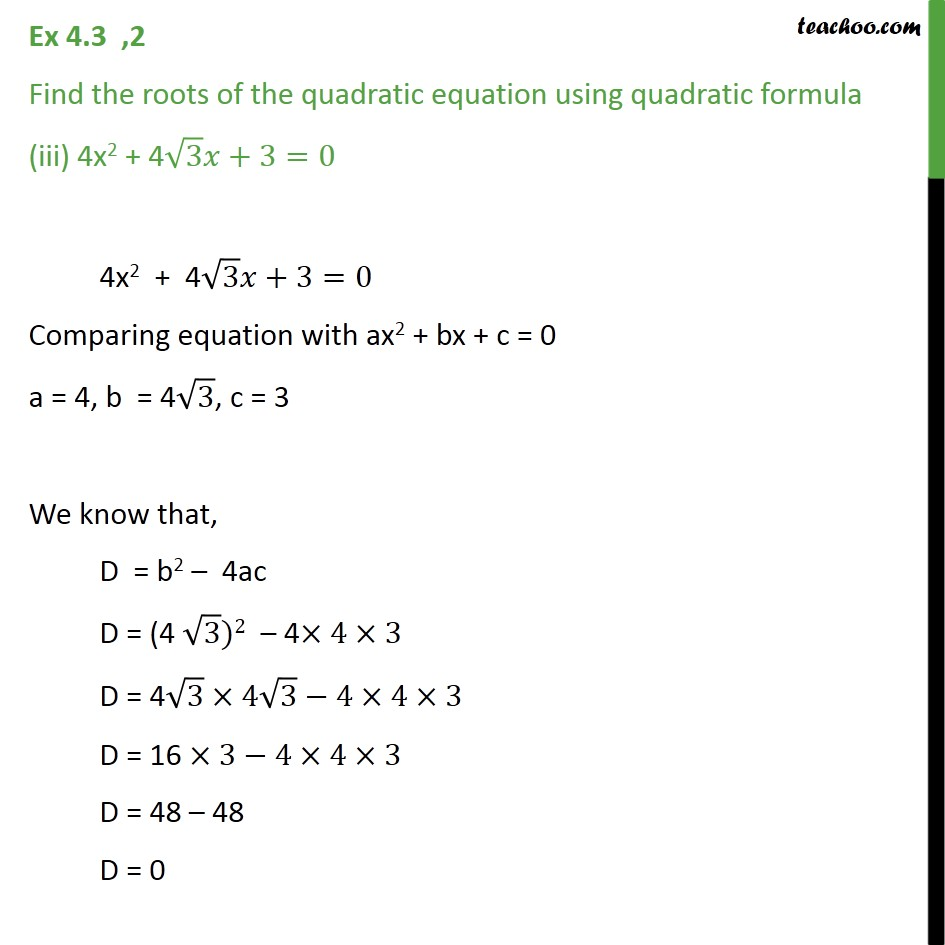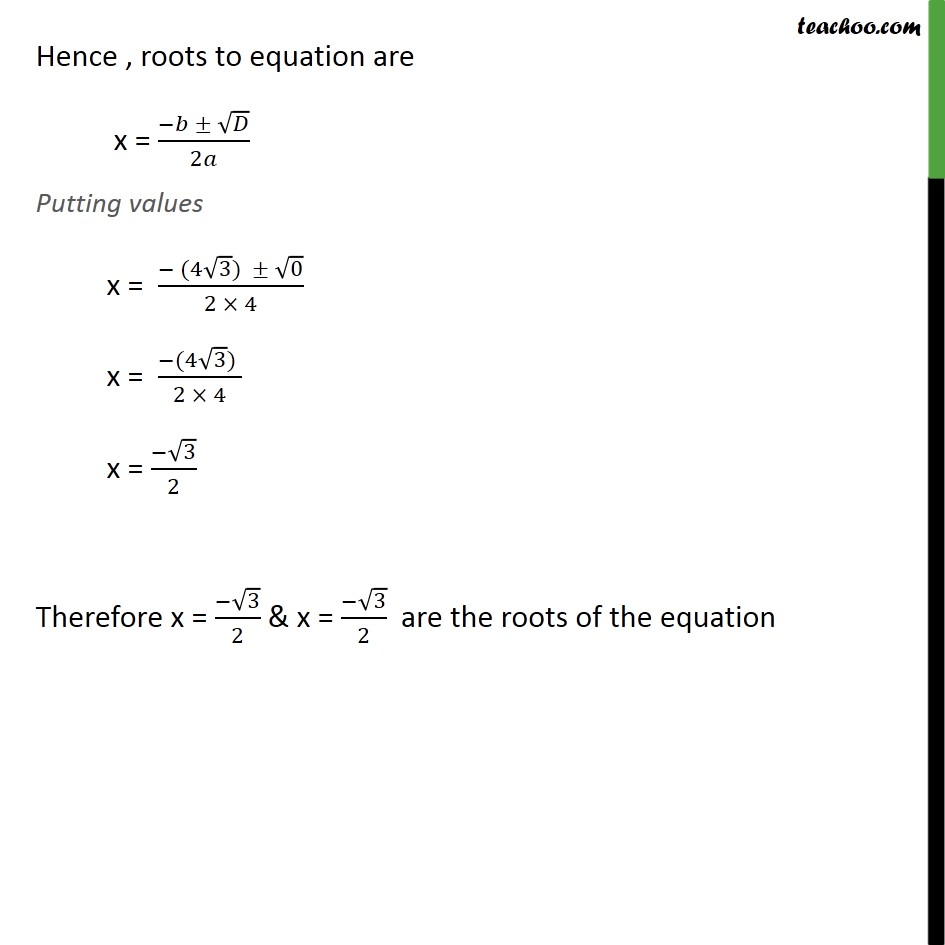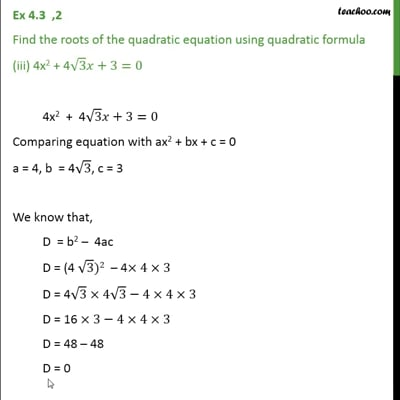Ex 4.3

Chapter 4 Class 10 Quadratic Equations
Serial order wiseThis video is only available for Teachoo black users

Learn in your speed, with individual attention - Teachoo Maths 1-on-1 Class

### Transcript

Ex 4.3 ,2 Find the roots of the quadratic equation using quadratic formula (iii) 4x2 + 4 3 +3=0 4x2 + 4 3 +3=0 Comparing equation with ax2 + bx + c = 0 a = 4, b = 4 3, c = 3 We know that, D = b2 4ac D = (4 3)2 4 4 3 D = 4 3 4 3 4 4 3 D = 16 3 4 4 3 D = 48 48 D = 0 Hence , roots to equation are x = ( )/2 Putting values x = ( (4 3) 0)/(2 4) x = ( (4 3) )/(2 4) x = ( 3)/2 Therefore x = ( 3)/2 & x = ( 3)/2 are the roots of the equation